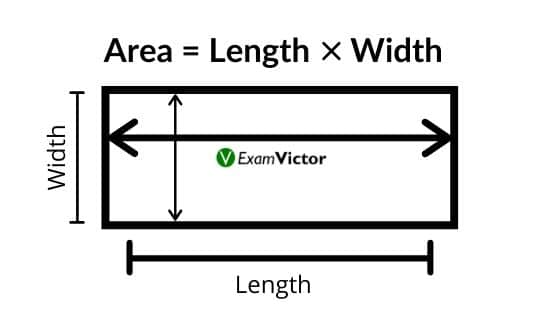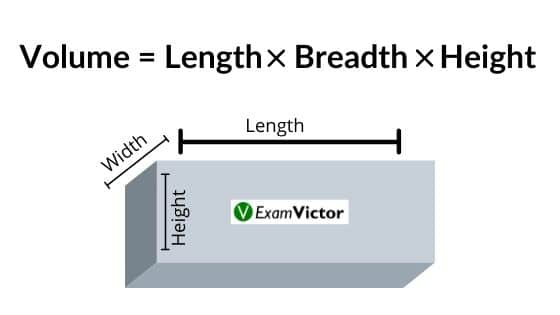# Basic Concepts of MensurationLast Updated on Nov 4, 2020

Mensuration is the skill of measuring the length of lines, areas of surfaces, and volumes of solids from simple data of lines and angles. Mensuration in its literal meaning is to measure. It is generally used where geometrical figures are concerned, where one has to determine various physical quantities such as perimeter, area, volume or length. Measuring these quantities is called Mensuration.

### Area:

Area is a quantity that expresses the extent of a two-dimensional surface or shape in the plane. Area can be understood as the amount of material with a given thickness that would be necessary to fashion a model of the shape, or the amount of paint necessary to cover the surface with a single coat. This is measured in square unit like cm², m², etc.

• Area of Rectangle: Length X Breadth
• Area of Triangle: 0.5 X Base X Height
• Area of Square: Side X Side (or Side^2)
• Surface Area of a Cylinder = 2 X Pi X Radius X (Radius + Height)
• Surface Area of a Sphere = 4 X Pi X Radius X Radius
• Surface Area of a Cube = 6 X Side X Side
• Surface Area of a Cuboid = 2 X (Length X Breadth + Breadth X Height + Length X Height)Area of Rectangle

### Volume:

It refers to the quantity of three-dimensional space enclosed by a closed surface, for example, the space that a substance (solid, liquid, gas, or plasma) or shape occupies or contains. Volume is often quantified numerically using the SI derived unit, the cubic meter. Given below are some standard definitions for volumes of recognizable objects:-

• Volume: Volume is the amount of space enclosed by a shape or object, how much 3-dimensional space (length, width, and height) it occupies. This is measured in cubic unit like cm³, m³, etc.
• Volume of Cuboid: Length X Breadth X Height
• Volume of Cube: Side X Side X Side
• Volume of Prism: Area of Base X Height
• Volume of Cone: 1/3 X Pi X Radius X Radius X HeightVolume of Rectangle

### Perimeter:

A perimeter is a path that surrounds a two-dimensional shape. The term may be used either for the path or its length—it can be thought of as the length of the outline of a shape. The perimeter of a circle or ellipse is called its circumference. As mentioned before, since perimeter is nothing but a measure of length, it’s SI unit will be the meter itself. Given below are some formulae for perimeters of two-dimensional figures:-

• Perimeter of Circle: 2 X Pi X Radius
• Perimeter of Triangle: Side A + Side B + Side C
• Perimeter of Square: 4 X Side
• Perimeter of Rectangle: 2 X (Length + Breadth)

### Summary

In this post, we have provided the basics of Mensuration. The components like Area, Volume and Perimeter are also explained with formulas. Share your views about this post in the comment section below. For more related posts click here.